Courses

# 28 Year NEET Questions: Classification Of Elements & Periodicity In Properties

## 36 Questions MCQ Test Chemistry 31 Years NEET Chapterwise Solved Papers | 28 Year NEET Questions: Classification Of Elements & Periodicity In Properties

Description
This mock test of 28 Year NEET Questions: Classification Of Elements & Periodicity In Properties for NEET helps you for every NEET entrance exam. This contains 36 Multiple Choice Questions for NEET 28 Year NEET Questions: Classification Of Elements & Periodicity In Properties (mcq) to study with solutions a complete question bank. The solved questions answers in this 28 Year NEET Questions: Classification Of Elements & Periodicity In Properties quiz give you a good mix of easy questions and tough questions. NEET students definitely take this 28 Year NEET Questions: Classification Of Elements & Periodicity In Properties exercise for a better result in the exam. You can find other 28 Year NEET Questions: Classification Of Elements & Periodicity In Properties extra questions, long questions & short questions for NEET on EduRev as well by searching above.
QUESTION: 1

### Pauling’s electronegativity values for elements are useful in predicting 

Solution:

Pauling scale of electronegativity was helpful in predicting (i) Nature of bond between two atoms (ii) Stability of bond by calculating the difference in electronegativities polarity of bond can be calculated.

QUESTION: 2

### The electronic configuration of four elements are given below. Which elements does not belong to the same family as others ? 

Solution:

A. B. D are the configuration of Zn, Cd and Hg respectively which belongs to the same family. But c is the configuration of chlorine which belongs to halogen family.

QUESTION: 3

### In the periodic table, with the increase in atomic number, the metallic character of an element 

Solution:

Metallic character decreases in a period and increases in a group.

QUESTION: 4

Elements of which of the following groups will form anions most readily ? 

Solution:

Electron affinity values are high in case of halogen because halogens have seven electrons (ns2np5) in the valence shell, they have a strong tendency to acquire the nearest inert gas configuration by gaining an electron from the metallic atom and form halide ions easily.

QUESTION: 5

Which of the following sets has strongest tendency to form anions ? 

Solution:

N, O and F (p-block elements) are highly electronegative non metals and will have the strongest tendency to form anions by gaining electrons from metal atoms.

QUESTION: 6

One would expect proton to have very large

Solution:

Proton (H+) being very small in size would have very large hydration energy.

QUESTION: 7

Na+, Mg2+, Al3+ and Si4+ are isoelectronic. The order of their ionic size is 

Solution:

Amongst isoelectronic ions, the size of the cation decreases as the magnitude of the charge increases.

QUESTION: 8

If the atomic number of an element is 33, it will be placed in the periodic table in the

Solution:

The electronic configuration of element with atomic number 33 is 1s22s22p63s23p63d104s24p3
As, its last shell have five electrons
and hence, its group is 10 + 5 = 15th or V A.
Hence,it lies in the 15th group.

QUESTION: 9

In the periodic table from left to right in a period, the atomic volume 

Solution:

Atomic volume is the volume occupied by one gram of an element. Within a period from left to right, atomic volume first decreases and then increases due to increases of nuclear charge and increase in the number of electrons in the valence shell.

QUESTION: 10

Which electronic configuration of an element has abnormally high difference between second and third ionization energy ? 

Solution:

Abnormally high difference between 2nd and 3rd ionization energy means that the element has two valence electrons, i.e., configuration (d)

QUESTION: 11

One of the characteristic properties of non-metals is that they

Solution:

Non-metal like halogens are strongest oxidizing agents according to electrochemical series they form acidic oxides like SO2 they form anion by electron gain and they are highly electronegative.
(fluorine is most electronegative element of whole periodic table)

QUESTION: 12

The electronic configuration of an element is 1s2 2s2 2p6 3s2 3p3 . What is the atomic number of the element, which is just below the above element in the periodic table? 

Solution:

Atomic number of the given element is 15 and it belongs to 5th group. Therefore atomic number of the element below the above element = 15 + 18 = 33.

QUESTION: 13

The element, with atomic number 118, will be

Solution:

Electronic configuration of element with atomic number 118 will be [Rn]5f146d10 7s27p6. Since its elctronic configuration in the outer most orbit (ns2np6) resemble with that of inert or noble gases, therefore it will be noble gas element.

QUESTION: 14

Which one of the following ions will be the smallest in size? 

Solution:

Greater is the positive charge on atom, large is effective nuclear charge. Hence smaller is the size

QUESTION: 15

Which of the following does not represent the correct order of the properties indicated 

Solution:

In a period on moving from left to right ionic radii decreases.

(a) So order of cationic radii is Cr2+ > Mn2+  > Fe2+ > Ni2+ and

(b) Sc > Ti > Cr > Mn (correct order of atomic radii)

(c) For unpaired electrons Mn2+ (Five)  Ni2+ (Two) < Co2+ (Three)<Fe2+ (Four )

(d) For unpaired electrons Fe2+(Four) < Co2+ (Three) <Ni2+ (Two)<Cu2+(One)

QUESTION: 16

The first ionization potentials (eV) of Be and B respectively are 

Solution:

First ionisation potential of Be is greater than boron due to following configuration 4Be=1s2,2s2 5B=1s2,2s22p1 Order of attraction of electrons towards nucleus 2s>2p, so more amount of energy is required to remove the electron with 2sorbital in comparison to 2p orbital.

QUESTION: 17

Of the given electronic configurations for the elements, which electronic configuration indicates that there will be abnormally high difference in the second and third ionization energy for the element? 

Solution:

Mg = 1s2 22 2p6 3s2 After removing of 2 electron, the magnesium acquired noble gas configuration hence removing of 3rd electron will require large amount of energy.

QUESTION: 18

Correct order of first IP among following elements Be, B, C, N, O is 

Solution:

Be – 1s22s2; B – 1s22s22p1; C – 1s22s22p2; N – 1s22s22p3; O – 1s22s22p4. IP increases along the period. But IP of Be > B. Further IP of O < N because atoms with fully  or partly filled orbitals are most stable and hence have high ionisation energy.

QUESTION: 19

Which of the following order is wrong? 

Solution:

Along the period, I.P. generally increases but not regularly. Be and B are exceptions. First I.P. increases in moving from left to right in a period, but I.P. of B is lower than Be.

QUESTION: 20

An atom has electronic configuration 1s2 2s2 2p6 3s2 3p6 3d3 4s2, you will place it in which group?

Solution:

The electronic configuration clearly suggest that it is a d-block element (having contiguration (n – 1) d1– 10 ns0 – 2) which starts from III B and goes till II B. Hence with d3 configuration it would be classified in the group.

QUESTION: 21

Which of the following statements is true?         

Solution:

This is because of inter-electronic replusions between lone pairs.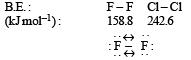QUESTION: 22

Among K, Ca, Fe and Zn the element which can form more than one binary compound with chlorine is 

Solution:

Among the given options, only Fe shows variable oxidation states so it can form two chlorides, viz. FeCl2 and FeCl3.

QUESTION: 23

Solution:

Ionic radii are inversely proportional to effective nuclear charge.
Ionic radii in the nth orbit is given as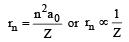when n = principal quantum number
Z-effective nuclear change.

QUESTION: 24

Which one of the following oxides is expected to exhibit paramagnetic behaviour? 

Solution:

Due to odd number of electr on s in ClO2.

Therefore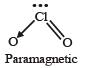QUESTION: 25

Which one of the following arrangements represents the correct order of electron gain enthalpy (with negative sign) of the given atomi c species? [2 00 5]

Solution:

The amount of energy released when an electron is added to an isolated gaseous atom to produce a monovalent anion is called electron gain enthalpy.
Electron affinity value generally increase on moving from left to right in a period however there are exceptions of this rule in the case of those atoms which have stable configuration. These atoms resist the addition of extra electron, therefore  the low value of electron affinity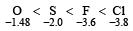On the other hand Cl because of its compariti very bigger size than F, allow the addition of an extra electron more easily.

QUESTION: 26

Identify the correct order of the size of the following: 

Solution:

For i soelectronic species, size of anion increases as negative charge increases whereas size of cation decreases with increase in positive charge. Further ionic radii of anions is more than that of cations. Thus the correct order is
Ca++ < K+ < Ar < Cl < S– –

QUESTION: 27

Which of the following electronic configuration an atom has the lowest ionisation enthalpy?

Solution:

The electronic configuration 1s2 2s22p5 3s1 shows lowest ionisation energy because this configuration is unstable due to the presence of one electron is s- orbital. Hence, less energy is required to remove the electron.

QUESTION: 28

Which one of the following ionic species has the greatest proton affinity to form stable compound?

Solution:

Proton affinity decreases in moving across the period from left to right due to increase in charge, within a group the proton affinities decreases from to p to bottom.
Nitrogen family > Oxygen family > Halogens

QUESTION: 29

The stability of + 1 oxidation state increases in the sequence: 

Solution:

The stability of +1 oxidation state increases from  aluminium to thallium i.e.
Al < Ga < In < Tl

Due to inert pair effect, as we move down the group. Lower oxidation state stablises so order of +1 oxidation state is:

Al < Ga < In < Tl

QUESTION: 30

Amongst the elements with following electronic configurations, which one of them may have the highest ionization energy? 

Solution:

The smaller the atomic size, larger is the value of ionisation potential. Further the atoms having half filled or fully filled orbitals are comparitively more stable, hence more energy is required to remove the electron from such atoms.

QUESTION: 31

The correct order of the decreasing ionic radii among the following isoelectronic species are :

Solution:

Among the isoelectronic species, size increases with the increase in negative charge. Thus S2– has the highest negative charge and hence largest in size followed by Cl, K+ and Ca.

QUESTION: 32

Which of the following represents the correct order of increasing electron gain enthalpy with negative sign for the elements O, S, F and Cl ? 

Solution:

O < S < F < CI

Electron gain enthalpy – 141 – 200 – 333 – 349 kJ mol–1

QUESTION: 33

Among the elements Ca, Mg, P and Cl, the order of increasing atomic radii is : 

Solution: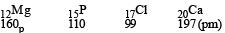QUESTION: 34

What is the value of electron gain enthalpy of Na+ if IE1 of Na = 5.1 eV ? [2011M]

Solution:

IE1 of Na = – Electron gain enthalpy of Na+                    = – 5.1 Volt.

QUESTION: 35

Identify the wrong statement in the following: 

Solution:

As the positive charge increases on metal cation, radius decreases. This is due to the fact that nuclear charge in the case of a cation is acting on lesser number of electrons and pulls them closer.

QUESTION: 36

Which one of the following arrangements represents the correct order of least negative to most negative electron gain enthalpy for C, Ca, Al, F and O? [NEET Kar. 2013]

Solution:

As the nuclear charge increases, the force of attraction between the nucleus and the incoming electron increses and hence the elecron gain enthalpy becomes more negative, hence the correct order is Ca   <   Al    <   C    <   O   <    F